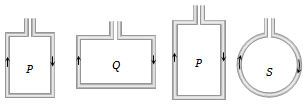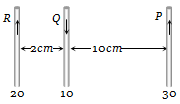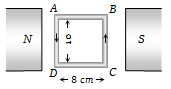An electron moves with a constant speed v along a circle of radius r. Its magnetic moment will be (e is the electron's charge)

(a) evr                        (b) $\frac{1}{2}$evr

(c) ${\mathrm{\pi r}}^{2}$ev                     (d) 2$\mathrm{\pi }$rev

Concept Questions :-

Magnetic moment
High Yielding Test Series + Question Bank - NEET 2020

Difficulty Level:

Four wires each of length 2.0 metres are bent into four loops P, Q, R and S and then suspended into uniform magnetic field. Same current is passed in each loop. Which statement is correct?(a) Couple on loop P will be the highest

(b) Couple on loop Q will be the highest

(c) Couple on loop R will be the highest

(d) Couple on loop S will be the highest

Concept Questions :-

Magnetic moment
High Yielding Test Series + Question Bank - NEET 2020

Difficulty Level:

A circular current carrying coil has a radius R. The distance from the centre of the coil on the axis where the magnetic induction will be $\frac{1}{8}\mathrm{th}$ to its value at the centre of the coil, is

(a) $\frac{\mathrm{R}}{\sqrt{3}}$                       (b)  $\mathrm{R}\sqrt{3}$

(c) $2\sqrt{3}\mathrm{R}$                     (d) $\frac{2}{\sqrt{3}}\mathrm{R}$

Concept Questions :-

Magnetic field due to various cases
High Yielding Test Series + Question Bank - NEET 2020

Difficulty Level:

The sensitivity of a moving coil galvanometer can be increased by decreasing

(a) The number of turns in the coil

(b) The area of the coil

(c) The magnetic field

(d) The couple per unit twist of the suspension

Concept Questions :-

Moving coil galvanometer
High Yielding Test Series + Question Bank - NEET 2020

Difficulty Level:

A metallic loop is placed in a magnetic field. If a current is passed through it, then

(a) The ring will feel a force of attraction

(b) The ring will feel a force of repulsion

(c) It will move to and fro about its centre of gravity

(d) None of these

Concept Questions :-

Current carrying loop : Force and torque
High Yielding Test Series + Question Bank - NEET 2020

Difficulty Level:

Three long, straight and parallel wires carrying currents are arranged as shown in figure. The force experienced by 10 cm length of wire Q is(a) $1.4×{10}^{-4}N$ towards the right

(b) $1.4×{10}^{-4}N$ towards the left

(c) $2.6×{10}^{-4}N$ to the right

(d) $2.6×{10}^{-4}N$ to the left

Concept Questions :-

Force between current carrying wires
High Yielding Test Series + Question Bank - NEET 2020

Difficulty Level:

A 100 turns coil shown in figure carries a current of 2 amp in a magnetic field $B=0.2Wb/{m}^{2}$. The torque acting on the coil is(a) 0.32 Nm tending to rotate the side AD out of the page

(b) 0.32 Nm tending to rotate the side AD into the page

(c) 0.0032 Nm tending to rotate the side AD out of the page

(d) 0.0032 Nm tending to rotate the side AD into the page

Concept Questions :-

Magnetic moment
High Yielding Test Series + Question Bank - NEET 2020

Difficulty Level:

A current of 5 amperes is flowing in a wire of length 1.5 meters. A force of 7.5 N acts on it when it is placed in a uniform magnetic field of 2 Tesla. The angle between the magnetic field and the direction of the current is:

1. 30$°$                     2. 45°

3. 60°                     4. 90°

Concept Questions :-

Lorentz force
High Yielding Test Series + Question Bank - NEET 2020

Difficulty Level:

The field normal to the plane of a wire of n turns and radius r which carries a current i is measured on the axis of the coil at a small distance h from the centre of the coil. This is smaller than the field at the centre by the fraction

(a) $\frac{3}{2}\frac{{\mathrm{h}}^{2}}{{\mathrm{r}}^{2}}$                       (b) $\frac{2}{3}\frac{{\mathrm{h}}^{2}}{{\mathrm{r}}^{2}}$

(c)  $\frac{3}{2}\frac{{\mathrm{r}}^{2}}{{\mathrm{h}}^{2}}$                       (d) $\frac{2}{3}\frac{{\mathrm{r}}^{2}}{{\mathrm{h}}^{2}}$

Concept Questions :-

Magnetic field due to various cases
High Yielding Test Series + Question Bank - NEET 2020

Difficulty Level:

In hydrogen atom, the electron is making $6.6×{10}^{15}rev/sec$ around the nucleus in an orbit of radius 0.528 Å. The magnetic moment $\left(A-{m}^{2}\right)$ will be

(a) $1×{10}^{-15}$                      (b) $1×{10}^{-10}$

(c) $1×{10}^{-23}$                       (d) $1×{10}^{-27}$

Concept Questions :-

Magnetic moment